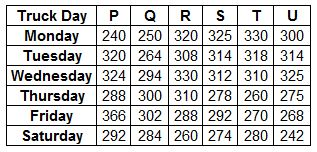### SBI Associate PO 2010 Question 141

Instructions

Study the table carefully to answer the question that follow:
Distance (in kms) travelled by six trucks on six different days of the weekQuestion 141

# What is the average distance travelled by truck S in all the days together?

Solution

Average distance travelled by truck S = $$\frac{325+314+312+278+292+274}{6} = 299\frac{1}{6}$$ km